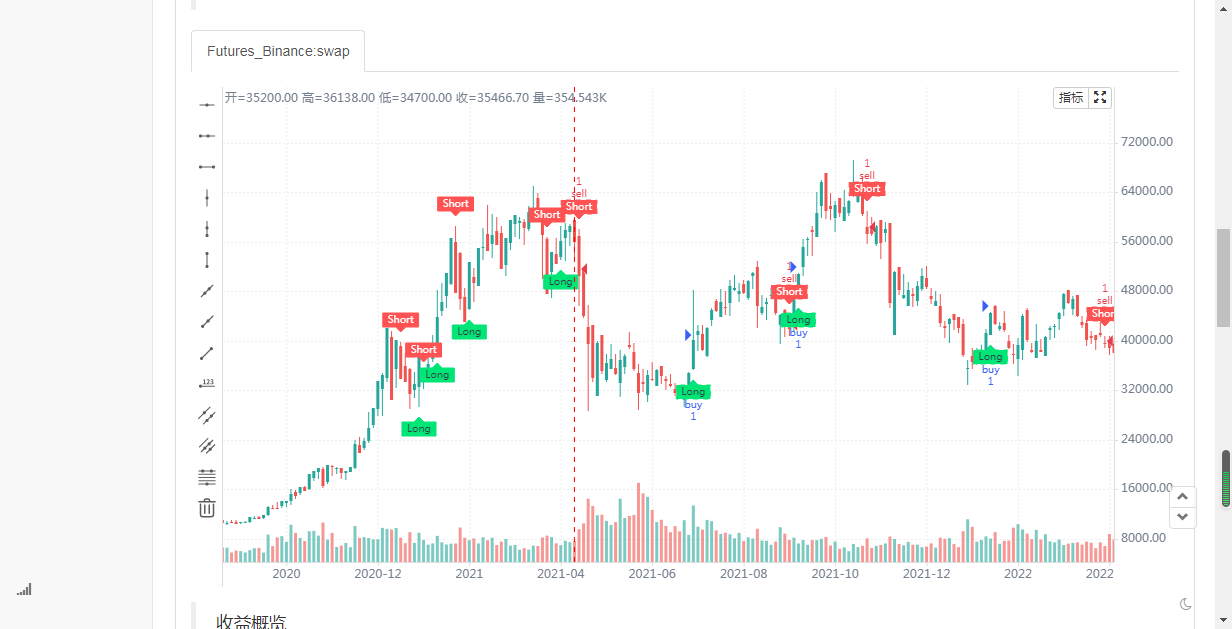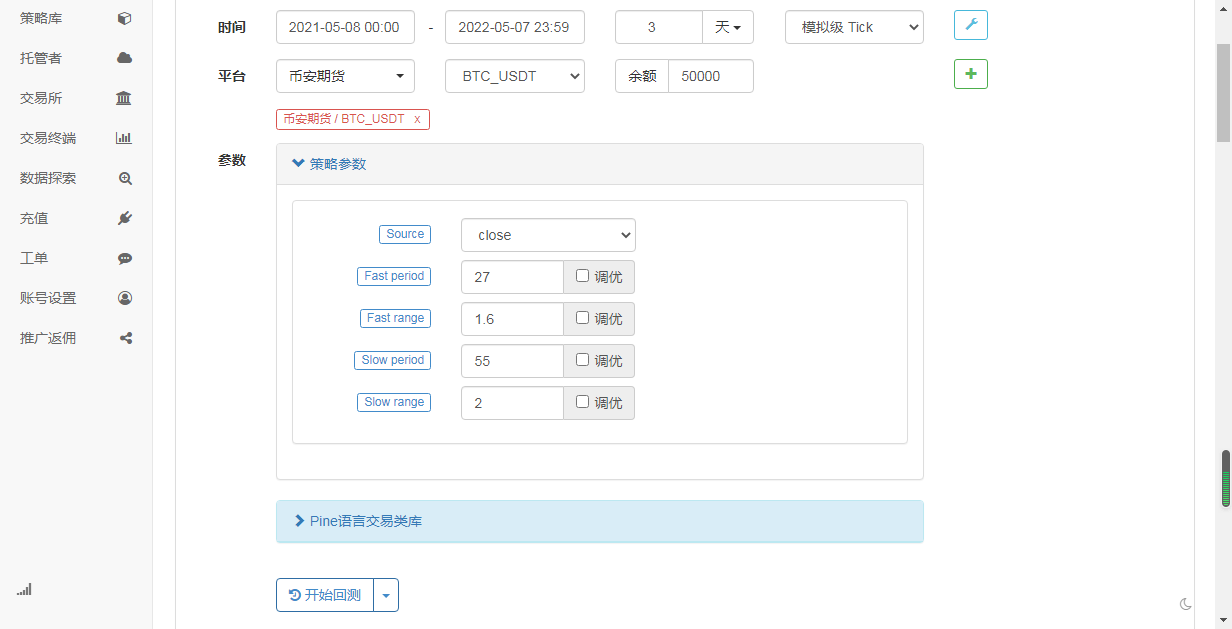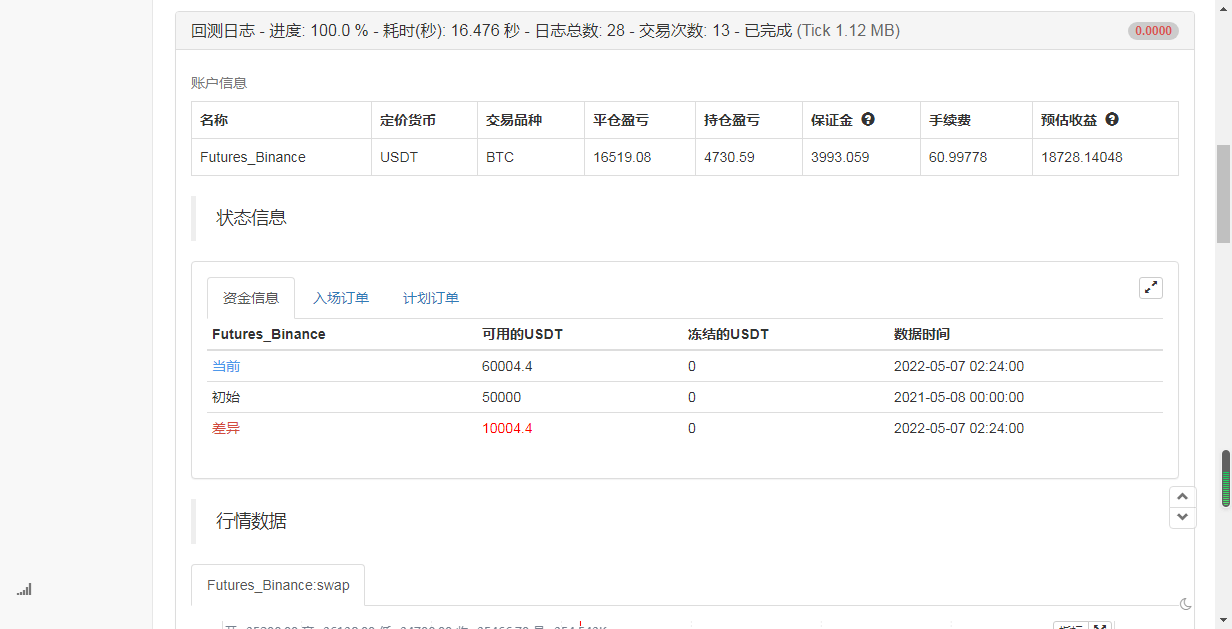# Twin Range Filter

Author: Zer3192, Date: 2022-06-04 20:24:49
Tags: EMA```/*backtest
start: 2021-05-08 00:00:00
end: 2022-05-07 23:59:00
period: 4h
basePeriod: 15m
exchanges: [{"eid":"Futures_Binance","currency":"BTC_USDT"}]
*/
// This source code is subject to the terms of the Mozilla Public License 2.0 at https://mozilla.org/MPL/2.0/

//@version=4

study(title="Twin Range Filter", overlay=true)

source = input(defval=close, title="Source")

// Smooth Average Range

per1 = input(defval=27, minval=1, title="Fast period")
mult1 = input(defval=1.6, minval=0.1, title="Fast range")

per2 = input(defval=55, minval=1, title="Slow period")
mult2 = input(defval=2, minval=0.1, title="Slow range")

smoothrng(x, t, m) =>
wper = t * 2 - 1
avrng = ema(abs(x - x), t)
smoothrng = ema(avrng, wper) * m
smoothrng
smrng1 = smoothrng(source, per1, mult1)
smrng2 = smoothrng(source, per2, mult2)
smrng = (smrng1 + smrng2) / 2

// Range Filter

rngfilt(x, r) =>
rngfilt = x
rngfilt := x > nz(rngfilt) ? x - r < nz(rngfilt) ? nz(rngfilt) : x - r :
x + r > nz(rngfilt) ? nz(rngfilt) : x + r
rngfilt
filt = rngfilt(source, smrng)

upward = 0.0
upward := filt > filt ? nz(upward) + 1 : filt < filt ? 0 : nz(upward)
downward = 0.0
downward := filt < filt ? nz(downward) + 1 : filt > filt ? 0 : nz(downward)

hband = filt + smrng
lband = filt - smrng

longCond = bool(na)
shortCond = bool(na)
longCond := source > filt and source > source and upward > 0 or source > filt and source < source and upward > 0
shortCond := source < filt and source < source and downward > 0 or source < filt and source > source and downward > 0

CondIni = 0
CondIni := longCond ? 1 : shortCond ? -1 : CondIni

long = longCond and CondIni == -1
short = shortCond and CondIni == 1

// Plotting

plotshape(long, title="Long", text="Long", style=shape.labelup, textcolor=color.black, size=size.tiny, location=location.belowbar, color=color.lime, transp=0)
plotshape(short, title="Short", text="Short", style=shape.labeldown, textcolor=color.white, size=size.tiny, location=location.abovebar, color=color.red, transp=0)